Test: Single Sideband (SSB) Modulation

# Test: Single Sideband (SSB) Modulation - Electronics and Communication Engineering (ECE)

Test Description

## 10 Questions MCQ Test GATE ECE (Electronics) 2023 Mock Test Series - Test: Single Sideband (SSB) Modulation

Test: Single Sideband (SSB) Modulation for Electronics and Communication Engineering (ECE) 2023 is part of GATE ECE (Electronics) 2023 Mock Test Series preparation. The Test: Single Sideband (SSB) Modulation questions and answers have been prepared according to the Electronics and Communication Engineering (ECE) exam syllabus.The Test: Single Sideband (SSB) Modulation MCQs are made for Electronics and Communication Engineering (ECE) 2023 Exam. Find important definitions, questions, notes, meanings, examples, exercises, MCQs and online tests for Test: Single Sideband (SSB) Modulation below.
Solutions of Test: Single Sideband (SSB) Modulation questions in English are available as part of our GATE ECE (Electronics) 2023 Mock Test Series for Electronics and Communication Engineering (ECE) & Test: Single Sideband (SSB) Modulation solutions in Hindi for GATE ECE (Electronics) 2023 Mock Test Series course. Download more important topics, notes, lectures and mock test series for Electronics and Communication Engineering (ECE) Exam by signing up for free. Attempt Test: Single Sideband (SSB) Modulation | 10 questions in 30 minutes | Mock test for Electronics and Communication Engineering (ECE) preparation | Free important questions MCQ to study GATE ECE (Electronics) 2023 Mock Test Series for Electronics and Communication Engineering (ECE) Exam | Download free PDF with solutions
 1 Crore+ students have signed up on EduRev. Have you?
Test: Single Sideband (SSB) Modulation - Question 1

### The power contained in single sideband in amplitude modulation is

Detailed Solution for Test: Single Sideband (SSB) Modulation - Question 1

Concept:

The total transmitted power for an AM system is given by: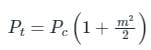Pc = Carrier Power

m = Modulation Index

Analysis:

The above expression can be expanded to get: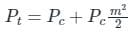The total power is the sum of the carrier power and the sideband power, i.e.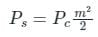In conventional AM transmission, the power is equally distributed between the two sidebands, i.e.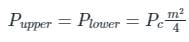∴ The power contained in a single sideband in amplitude modulation is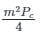Test: Single Sideband (SSB) Modulation - Question 2

### How much percentage power will be saved when the carrier and one of the sidebands are suppressed in an AM wave modulated to a depth of 50%?

Detailed Solution for Test: Single Sideband (SSB) Modulation - Question 2

Concept:

Total power in AM (PT)= PC ( 1 + μ2/2 ) = PC + PCμ2/2

where;

PC → Carrier power

μ → modulation index

Power of single sideband = ( PCμ2/2)/2 =  PCμ2/4

Calculation:

Given

μ = 50% = 0.5

After suppressing carrier and a single sideband :

PT = (PC + PCμ2/2) - (PC + PCμ2/4) = PCμ2/4

Percentage of power will be saved :

= [(PC + PCμ2/2 )- PCμ2/4 ]/PC ( 1 + μ2/2 ) × 100

= (1 + μ2/4)/( 1 + μ2/2 ) × 100

= (1 + (0.5)2/4)/( 1 + (0.5)2/2 ) × 100

94.44 %

Test: Single Sideband (SSB) Modulation - Question 3

### Pilot carrier in SSB modulation is used for

Detailed Solution for Test: Single Sideband (SSB) Modulation - Question 3

In single-sideband (SSB) modulation only the upper sideband or the lower sideband is transmitted (the carrier is suppressed).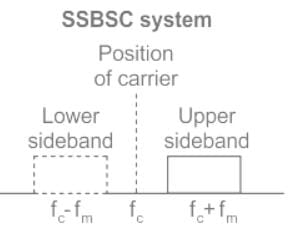• The major problem with the detection techniques in SSB is to have a coherent source at the receiver.
• If the frequency error becomes a function of time then it becomes difficult to recover the original signal.
• Since demodulation is hard in SSB signals, a low power carrier wave is transmitted with the SSB, which is called PILOT CARRIER.
•  This can be received and amplified at the receiving end to demodulate the SSB signal.
Test: Single Sideband (SSB) Modulation - Question 4

In the phase shift SSB method to get upper side band

Detailed Solution for Test: Single Sideband (SSB) Modulation - Question 4

Concept:

SSB (Single Side Band) modulation is a technique that allows only one sideband to pass saving bandwidth and power both. Practically it is very difficult to pass only a single sideband.

Analysis:

Ex: If we consider a single-tone modulating signal as x(t) = cos ωmt, the SSB modulated wave with only the upper sideband will be represented as:

cos ((ωc + ωm)t) = cos ωc.cos ωmt – sin ωc.sin ωmt

and with lower sideband only will be represented as:

cos (ωc - ωm)t = cos ωct .cos ωmt + sin ωct. sin ωmt

where sin ωmt is the Hilbert transform of the original message signal x(t) = cos ωmt

Conclusion:

Generalizing this for any signal m(t), we can say that the SSB modulated signal with only upper sideband will be:

m(t) cos ωct – m̂(t) sin ωct,

Similarly, we can say that the SSB modulated signal with only lower sideband will be:

m(t) cos ωct + m̂(t) sin ωct,

where m̂(t) is the Hilbert transform of m(t).

SSB/SC is generated using a balanced modulator circuit as shown: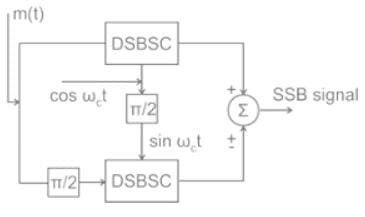Where π/2 phase shifter gives the Hilbert transform of the incoming signal.

Test: Single Sideband (SSB) Modulation - Question 5

Which of the following modulation scheme is most bandwidth efficient?

Detailed Solution for Test: Single Sideband (SSB) Modulation - Question 5

The bandwidth of different modulation schemes are as shown:

AM → 2fm

SSB-SC → fm

For angle modulated schemes (FM and PM)

= 2(β + 1) fm

fm = message signal bandwidth

So, for SSB-SC, the bandwidth requirement is minimal.

Test: Single Sideband (SSB) Modulation - Question 6

Which of the following circuits could NOT be used as demodulator of SSB?

Detailed Solution for Test: Single Sideband (SSB) Modulation - Question 6

Phase Discrimination is used for the demodulation of Frequency Modulate signals.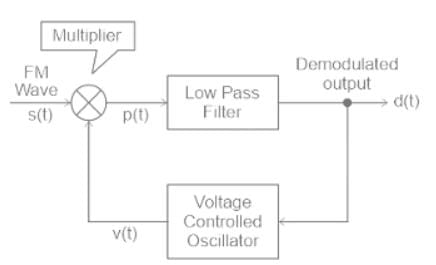• The following methods are used for the demodulation of Single Side Band (SSB) signals.
• The Filter Method
• The Phasing Shift Method
• The Weaver Demodulator
• Fourier based Demodulation.
• The following methods are used for the demodulation of Frequency Modulated (FM) signals.
• ​Frequency discrimination method
• Phase discrimination method.

​Hence, phase discriminator is used as demodulator of SSB

Test: Single Sideband (SSB) Modulation - Question 7

Direction: Two statements are given. Based on the given information, choose the correct answer.

Statement-I: For the same message signal, the pre-detection SNR is double in the case of conventional practical AM modulation, than that in the case of SSB.

Statement-II: The AM signal bandwidth is double as compared to SSB signal bandwidth.

Detailed Solution for Test: Single Sideband (SSB) Modulation - Question 7

Let, the channel is practical having finite attenuation of 'A' dB, and the single-sided noise power spectral density is given as 'N' W/Hz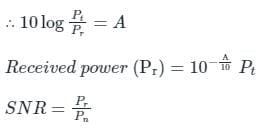Let the message signal spectrum is given as: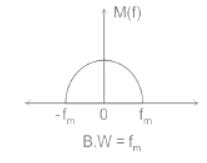In the case of AM:-

B.W = 2fm kHz (twice of message signal bandwidth)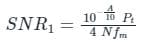In the case of SSB:-

B.W = fm kHz (same as message signal bandwidth)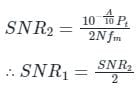∴ SNR in the case of S.S.B is double that in the case of AM.

Statement-I is incorrect and Statement-II is correct.

Test: Single Sideband (SSB) Modulation - Question 8

What should be the bandwidth figure of signal to noise ratio for SSB?

Detailed Solution for Test: Single Sideband (SSB) Modulation - Question 8

Concept:

SSB (Single Side Band) modulation is a technique that allows only one sideband to pass saving bandwidth and power both.

Practically it is very difficult to pass only a single sideband.

Ex: If we consider a single-tone modulating signal as x(t) = cos ωmt, the SSB modulated wave with only the upper sideband will be represented as:
cos ((ωc + ωm)t) = cos ωc.cos ωmt – sin ωc.sin ωmt

and with lower sideband only will be represented as:

cos (ωc - ωm)t = cos ωct .cos ωmt + sin ωct. sin ωmt

where sin ωmt is the Hilbert transform of the original message signal x(t) = cos ωmt

Generalizing this for any signal m(t), we can say that the SSB modulated signal with only upper sideband will be:
m(t) cos ωct – m̂(t) sin ωct,

Similarly, we can say that the SSB modulated signal with only lower sideband will be:

m(t) cos ωct + m̂(t) sin ωct

where m̂(t) is the Hilbert transform of m(t).

The generalized AM expression is represented as:

s(t) = Ac [1 + μmn (t)] cos ωct

The RF bandwidth of an SSB-AM signal is also 3 kHz.

Test: Single Sideband (SSB) Modulation - Question 9

The signal m(t) = cos(ωmt) is SSB (single side-band) modulated with a carrier cos(ωct) to get s(t). The signal obtained by passing s(t) through an ideal envelope detector is

Detailed Solution for Test: Single Sideband (SSB) Modulation - Question 9

1) General equation of SSB modulated wave: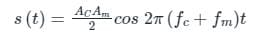2) Output of envelope detector to signal

Acos ωct → A

Application:

Equation of SSB modulated signal

Ac = Am = 1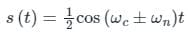the output of envelope detector = ½

The output of the envelope detector is a constant voltage signal

Hence option (d) which represents a constant voltage is correct.

Test: Single Sideband (SSB) Modulation - Question 10

The modulation technique that takes the lowest bandwidth among the given

Detailed Solution for Test: Single Sideband (SSB) Modulation - Question 10

The modulation technique that takes the lowest bandwidth is SSB-SC

Single Side Band (SSB SC):

1) SSB SC is a form of amplitude modulation where only a single sideband is transmitted and 1 sideband and carrier are suppressed.

2) The advantage of SSB is bandwidth and power-saving over both conventional AM and DSB SC, i.e. DSB SC has a more power consumption than SSB SC.

3) The Bandwidth requirement is only fm, ∴ It needs half the bandwidth than the DSB-SC transmission.

Comparison: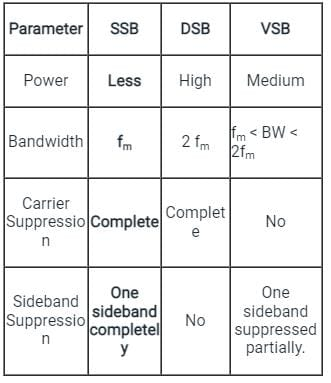## GATE ECE (Electronics) 2023 Mock Test Series

21 docs|263 tests
Information about Test: Single Sideband (SSB) Modulation Page
In this test you can find the Exam questions for Test: Single Sideband (SSB) Modulation solved & explained in the simplest way possible. Besides giving Questions and answers for Test: Single Sideband (SSB) Modulation, EduRev gives you an ample number of Online tests for practice

## GATE ECE (Electronics) 2023 Mock Test Series

21 docs|263 tests(Scan QR code)